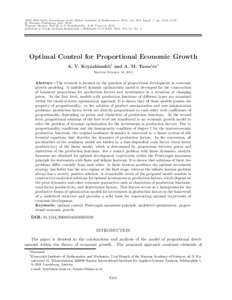# Optimal control for proportional economic growth

Kryazhimskii, A.V. & Tarasyev, A.M. (2016). Optimal control for proportional economic growth. Proceedings of the Steklov Institute of Mathematics 293 (S1) 101-119. 10.1134/S0081543816050102.Preview
Text
art%3A10.1134%2FS0081543816050102.pdf - Supplemental Material

## Abstract

The research is focused on the question of proportional development in economic growth modeling. A multilevel dynamic optimization model is developed for the construction of balanced proportions for production factors and investments in a situation of changing prices. At the first level, models with production functions of different types are examined within the classical static optimization approach. It is shown that all these models possess the property of proportionality: in the solution of product maximization and cost minimization problems, production factor levels are directly proportional to each other with coefficients of proportionality depending on prices and elasticities of production functions. At the second level, proportional solutions of the first level are transferred to an economic growth model to solve the problem of dynamic optimization for the investments in production factors. Due to proportionality conditions and the homogeneity condition of degree 1 for the macroeconomic production functions, the original nonlinear dynamics is converted to a linear system of differential equations that describe the dynamics of production factors. In the conversion, all peculiarities of the nonlinear model are hidden in a time-dependent scale factor (total factor productivity) of the linear model, which is determined by proportions between prices and elasticities of the production functions. For a control problem with linear dynamics, analytic formulas are obtained for optimal development trajectories within the Pontryagin maximum principle for statements with finite and infinite horizons. It is shown that solutions of these two problems differ crucially from each other: in finite horizon problems the optimal investment strategy inevitably has the zero regime at the final stage, whereas the infinite horizon problem always has a strictly positive solution. A remarkable result of the proposed model consists in constructive analytical solutions for optimal investments in production factors, which depend on the price dynamics and other economic parameters such as elasticities of production functions, total factor productivity, and depreciation factors. This feature serves as a background for the productive fusion of optimization models for investments in production factors in the framework of a multilevel structure and provides a solid basis for constructing optimal trajectories of economic development.

Item Type: Article Original Russian Text © A.V. Kryazhimskii, A.M. Taras’ev, 2015, published in Trudy Instituta Matematiki i Mekhaniki UrO RAN, 2015, Vol. 21, No. 2. optimal control; Pontryagin maximum principle; multilevel optimization; proportional economic growth Advanced Systems Analysis (ASA) Romeo Molina 12 Dec 2016 15:54 27 Aug 2021 17:41 http://pure.iiasa.ac.at/14119View Item